Latest Banking jobs   »   Quantitative Aptitude Quiz For SBI Clerk...

# Quantitative Aptitude Quiz For SBI PO Mains 2023- 16th January

Directions (1-4): Given below line graph shows total number of students( boys + girls)) in five different years in a college ‘X’ ,also shows total number of girls. Read the data carefully and answer the questions.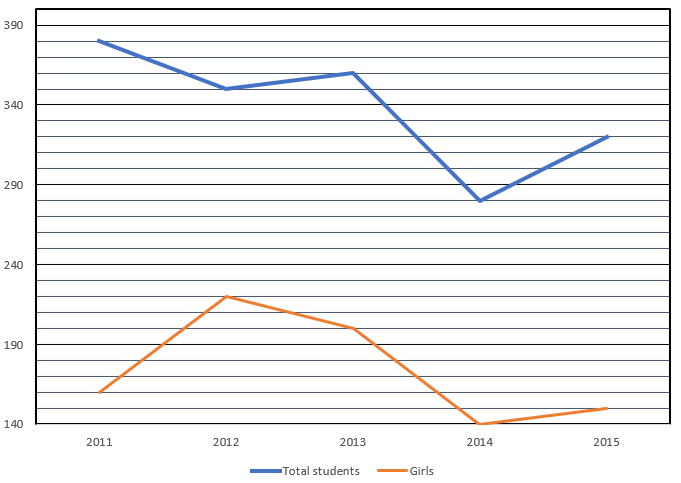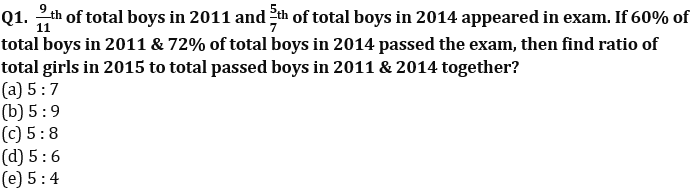Q2. In 2016 in college X, total students increased by 25% over the year 2015 and total students in college ‘Y’ in 2016 are 40% more than that of total students in college ‘X’ in same year. If sum of girls in the both colleges are 540 and total boys in college ‘X’ are 20 more than total boys in college ‘Y’ and each boy annual fee in college ‘Y’ in the year 2016 is Rs. 12000, then find total amount boys paid in college ‘Y’(in lakh Rs.)?
(a) 24
(b) 22
(c) 20
(d) 18
(e) None of these

Q3. Sum of total girls in the year 2015, 2016 & 2017 is 600 and total boys in the year 2015 & 2017 together is 100 more than total boys in the year 2016. If total girls in 2016, 2017 is 60% & 70% of total students in college, then find total boys in 2017 is what percent less than total boys in 2016?
(a) 41.25%
(b) 43.75%
(c) 43.25%
(d) 44.75%
(e) 42.25%

Q4. Average number of boys in the given years in college are what percent more than total boys in the year 2013?
(a) 1.5%
(b) 2%
(c) 1%
(d) 2.5%
(e) 3.5%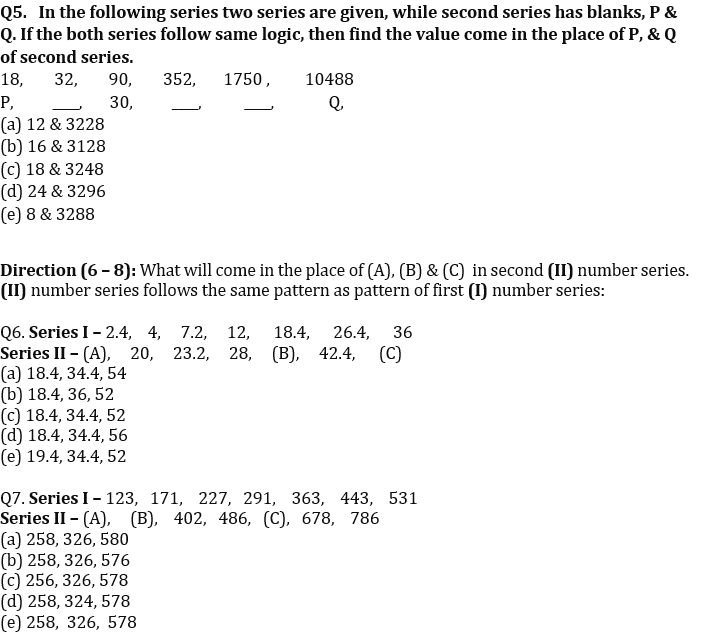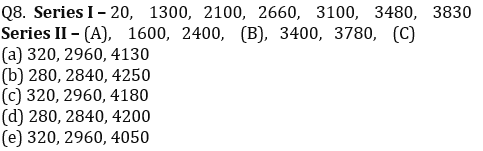Q9. If one series is 4.5, 12, 40, 165, 831, 4993 and another series which follows same pattern as given series is 7.5, __, __, __, __, a, then find value of 2a.
(a) 14308
(b) 7153
(c) 14306
(d) 14310
(e) 14318

Q10. If one series is 1880, 1640, 1448, 1304, 1208, 1160 and another series which follows same pattern as given series, then what will be 5th term of new formed series which starts with 2640?
(a) 1964
(b) 1978
(c) 1972
(d) 1968
(e) 1984

Q11. X invested Rs. P at 10% per annum, Y invested Rs.(P – 4000) less amount than X at the rate of 12% per annum. If they both get total compound interest at the end of two years Rs. 6412.8, then find capital invested by Y ?
(a) 16000 Rs.
(b) 12000 Rs.
(c) 10000 Rs.
(d) 14000 Rs
(e) 18000 Rs.

Q12. B is 40% less efficient than A and efficiency of C is ¼th the efficiency of A and B together. If C joined A and B every third day, then all three together complete the work in 27¾ days. Find in how many days will B alone complete the work?
(a) 40days
(b) 60days
(c) 50days
(d) 100days
(e) 80days

Q13.Ratio of Vodka and Wine in vessel A is 5 : 3 and same mixture in vessel B in the ratio of 3 : 2, 16ℓ of mixture from vessel A taken out and poured in vessel B new ratio of Vodka to wine becomes 29 : 19. If new quantity of mixture in vessel B is equal to initial quantity of mixture in vessel A, then find quantity of Vodka after 16 ℓ of mixture has been taken out from vessel A ?
(a) 50l
(b) 48l
(c) 54l
(d) 80l
(e) 84l

Q14. A boat takes double times in covering same distance in upstream that of downstream, if boat cover 96 km in downstream and 72 upstream in total 20 hours. Find still water speed of boat ?
(a) 6km/hr
(b) 8km/hr
(c) 7km/hr
(d) 9 km/hr
(e) 12km/hr

Q15. A train P with speed 64 km/hr crosses a pole in 27 second and an another train Q whose speed is 12½% less than train P crosses a man in 36 second. If both train move in opposite direction to each other, then find in how much time will they cross each other?
(a) 28.2 sec
(b) 36.2 sec
(c) 31.2 sec
(d) 38.2sec
(e) 39.2 sec

Solutions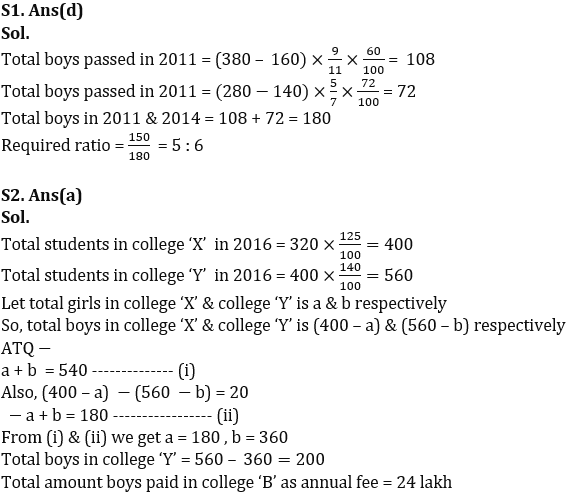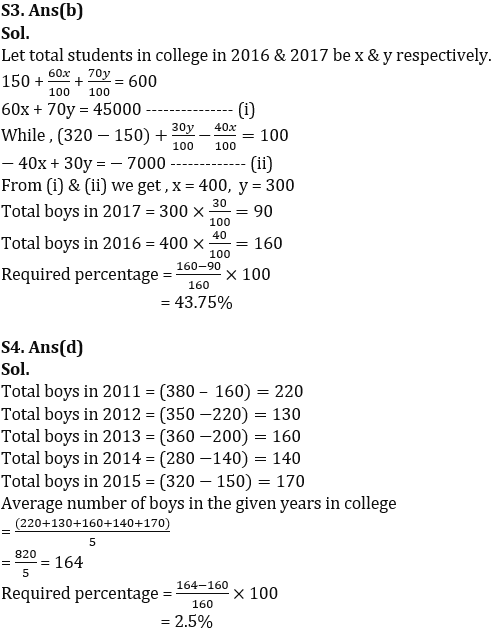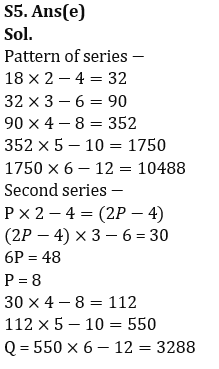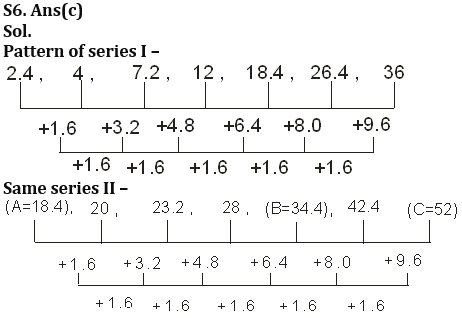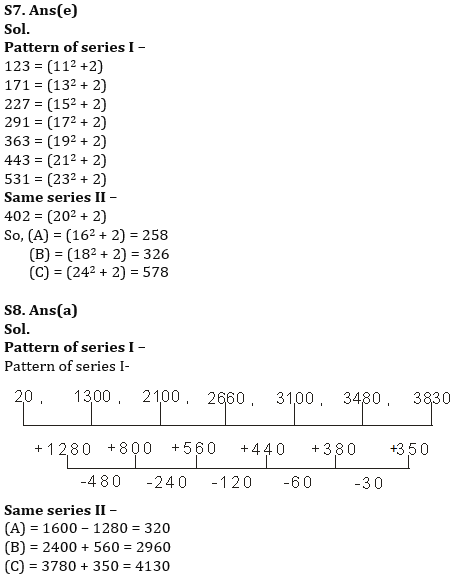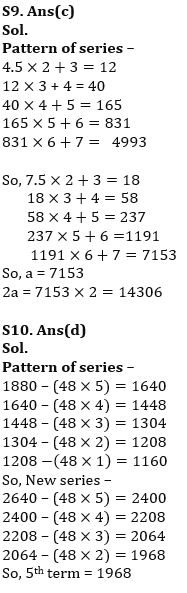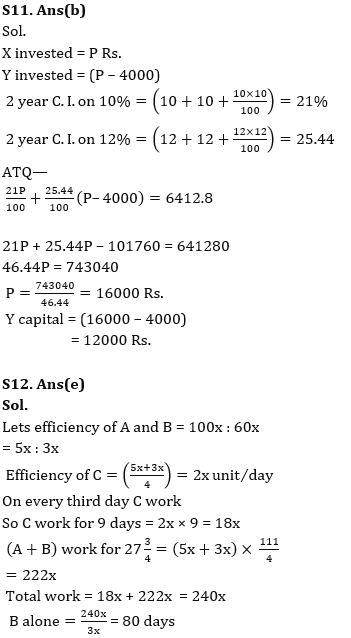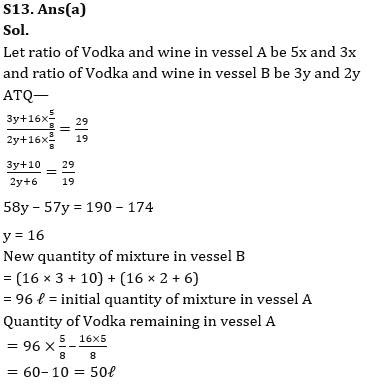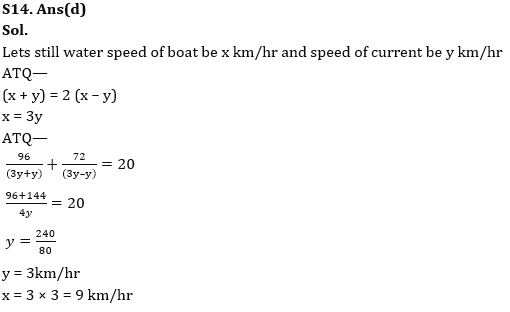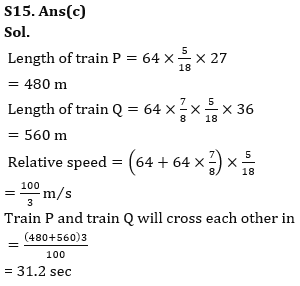## FAQs

### Q1. 9/11th of total boys in 2011 and 5/7th of total boys in 2014 appeared in exam. If 60% of total boys in 2011 & 72% of total boys in 2014 passed the exam, then find ratio of total girls in 2015 to total passed boys in 2011 & 2014 together?

S1. Ans(d)

#### Congratulations!Union Budget 2023-24: Free PDF